Newton’s Laws of Motion

# Forces

### Learning Objectives

By the end of the section, you will be able to:

• Distinguish between kinematics and dynamics
• Understand the definition of force
• Identify simple free-body diagrams
• Define the SI unit of force, the newton
• Describe force as a vector

The study of motion is called kinematics, but kinematics only describes the way objects move—their velocity and their acceleration. Dynamics is the study of how forces affect the motion of objects and systems. It considers the causes of motion of objects and systems of interest, where a system is anything being analyzed. The foundation of dynamics are the laws of motion stated by Isaac Newton (1642–1727). These laws provide an example of the breadth and simplicity of principles under which nature functions. They are also universal laws in that they apply to situations on Earth and in space.

Newton’s laws of motion were just one part of the monumental work that has made him legendary ((Figure)). The development of Newton’s laws marks the transition from the Renaissance to the modern era. Not until the advent of modern physics was it discovered that Newton’s laws produce a good description of motion only when the objects are moving at speeds much less than the speed of light and when those objects are larger than the size of most molecules (about ${10}^{-9}$ m in diameter). These constraints define the realm of Newtonian mechanics. At the beginning of the twentieth century, Albert Einstein (1879–1955) developed the theory of relativity and, along with many other scientists, quantum mechanics. Quantum mechanics does not have the constraints present in Newtonian physics. All of the situations we consider in this chapter, and all those preceding the introduction of relativity in Relativity, are in the realm of Newtonian physics.

Isaac Newton (1642–1727) published his amazing work, Philosophiae Naturalis Principia Mathematica, in 1687. It proposed scientific laws that still apply today to describe the motion of objects (the laws of motion). Newton also discovered the law of gravity, invented calculus, and made great contributions to the theories of light and color.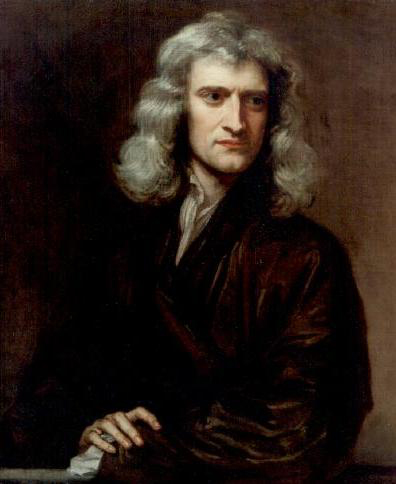### Working Definition of Force

Dynamics is the study of the forces that cause objects and systems to move. To understand this, we need a working definition of force. An intuitive definition of force—that is, a push or a pull—is a good place to start. We know that a push or a pull has both magnitude and direction (therefore, it is a vector quantity), so we can define force as the push or pull on an object with a specific magnitude and direction. Force can be represented by vectors or expressed as a multiple of a standard force.

The push or pull on an object can vary considerably in either magnitude or direction. For example, a cannon exerts a strong force on a cannonball that is launched into the air. In contrast, Earth exerts only a tiny downward pull on a flea. Our everyday experiences also give us a good idea of how multiple forces add. If two people push in different directions on a third person, as illustrated in (Figure), we might expect the total force to be in the direction shown. Since force is a vector, it adds just like other vectors. Forces, like other vectors, are represented by arrows and can be added using the familiar head-to-tail method or trigonometric methods. These ideas were developed in Vectors.

(a) An overhead view of two ice skaters pushing on a third skater. Forces are vectors and add like other vectors, so the total force on the third skater is in the direction shown. (b) A free-body diagram representing the forces acting on the third skater.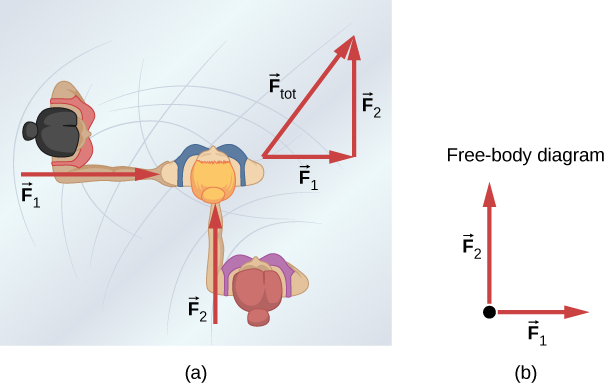(Figure)(b) is our first example of a free-body diagram, which is a sketch showing all external forces acting on an object or system. The object or system is represented by a single isolated point (or free body), and only those forces acting on it that originate outside of the object or system—that is, external forces—are shown. (These forces are the only ones shown because only external forces acting on the free body affect its motion. We can ignore any internal forces within the body.) The forces are represented by vectors extending outward from the free body.

Free-body diagrams are useful in analyzing forces acting on an object or system, and are employed extensively in the study and application of Newton’s laws of motion. You will see them throughout this text and in all your studies of physics. The following steps briefly explain how a free-body diagram is created; we examine this strategy in more detail in Drawing Free-Body Diagrams.

Problem-Solving Strategy: Drawing Free-Body Diagrams
1. Draw the object under consideration. If you are treating the object as a particle, represent the object as a point. Place this point at the origin of an xy-coordinate system.
2. Include all forces that act on the object, representing these forces as vectors. However, do not include the net force on the object or the forces that the object exerts on its environment.
3. Resolve all force vectors into x– and y-components.
4. Draw a separate free-body diagram for each object in the problem.

We illustrate this strategy with two examples of free-body diagrams ((Figure)). The terms used in this figure are explained in more detail later in the chapter.

In these free-body diagrams, $\stackrel{\to }{N}$ is the normal force, $\stackrel{\to }{w}$ is the weight of the object, and $\stackrel{\to }{f}$ is the friction.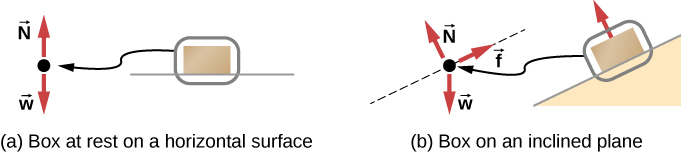The steps given here are sufficient to guide you in this important problem-solving strategy. The final section of this chapter explains in more detail how to draw free-body diagrams when working with the ideas presented in this chapter.

### Development of the Force Concept

A quantitative definition of force can be based on some standard force, just as distance is measured in units relative to a standard length. One possibility is to stretch a spring a certain fixed distance ((Figure)) and use the force it exerts to pull itself back to its relaxed shape—called a restoring force—as a standard. The magnitude of all other forces can be considered as multiples of this standard unit of force. Many other possibilities exist for standard forces. Some alternative definitions of force will be given later in this chapter.

The force exerted by a stretched spring can be used as a standard unit of force. (a) This spring has a length x when undistorted. (b) When stretched a distance $\text{Δ}x$, the spring exerts a restoring force ${\stackrel{\to }{F}}_{\text{restore}},$ which is reproducible. (c) A spring scale is one device that uses a spring to measure force. The force ${\stackrel{\to }{F}}_{\text{restore}}$ is exerted on whatever is attached to the hook. Here, this force has a magnitude of six units of the force standard being employed.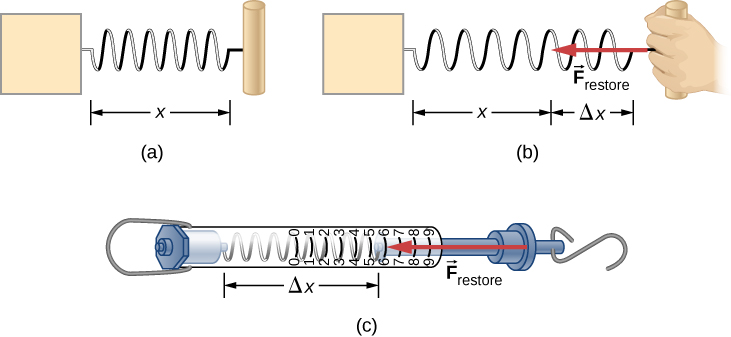Let’s analyze force more deeply. Suppose a physics student sits at a table, working diligently on his homework ((Figure)). What external forces act on him? Can we determine the origin of these forces?

(a) The forces acting on the student are due to the chair, the table, the floor, and Earth’s gravitational attraction. (b) In solving a problem involving the student, we may want to consider the forces acting along the line running through his torso. A free-body diagram for this situation is shown.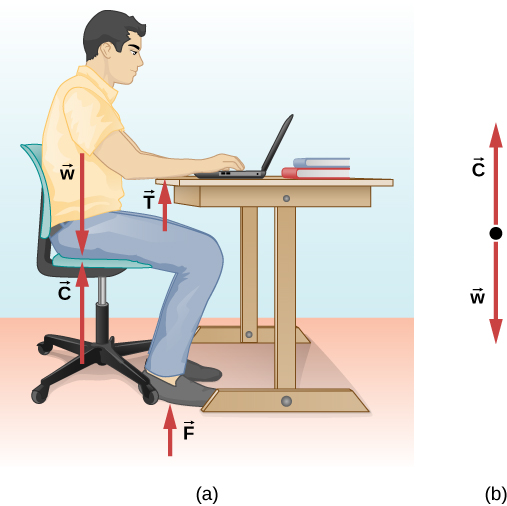In most situations, forces are grouped into two categories: contact forces and field forces. As you might guess, contact forces are due to direct physical contact between objects. For example, the student in (Figure) experiences the contact forces $\stackrel{\to }{C}$, $\stackrel{\to }{F}$, and $\stackrel{\to }{T}$, which are exerted by the chair on his posterior, the floor on his feet, and the table on his forearms, respectively. Field forces, however, act without the necessity of physical contact between objects. They depend on the presence of a “field” in the region of space surrounding the body under consideration. Since the student is in Earth’s gravitational field, he feels a gravitational force $\stackrel{\to }{w}$; in other words, he has weight.

You can think of a field as a property of space that is detectable by the forces it exerts. Scientists think there are only four fundamental force fields in nature. These are the gravitational, electromagnetic, strong nuclear, and weak fields (we consider these four forces in nature later in this text). As noted for $\stackrel{\to }{w}$ in (Figure), the gravitational field is responsible for the weight of a body. The forces of the electromagnetic field include those of static electricity and magnetism; they are also responsible for the attraction among atoms in bulk matter. Both the strong nuclear and the weak force fields are effective only over distances roughly equal to a length of scale no larger than an atomic nucleus (${10}^{-15}\phantom{\rule{0.2em}{0ex}}\text{m}$). Their range is so small that neither field has influence in the macroscopic world of Newtonian mechanics.

Contact forces are fundamentally electromagnetic. While the elbow of the student in (Figure) is in contact with the tabletop, the atomic charges in his skin interact electromagnetically with the charges in the surface of the table. The net (total) result is the force $\stackrel{\to }{T}$. Similarly, when adhesive tape sticks to a piece of paper, the atoms of the tape are intermingled with those of the paper to cause a net electromagnetic force between the two objects. However, in the context of Newtonian mechanics, the electromagnetic origin of contact forces is not an important concern.

### Vector Notation for Force

As previously discussed, force is a vector; it has both magnitude and direction. The SI unit of force is called the newton (abbreviated N), and 1 N is the force needed to accelerate an object with a mass of 1 kg at a rate of $1\phantom{\rule{0.2em}{0ex}}{\text{m/s}}^{2}$: $1\phantom{\rule{0.2em}{0ex}}\text{N}=1\phantom{\rule{0.2em}{0ex}}\text{kg}·{\text{m/s}}^{2}.$ An easy way to remember the size of a newton is to imagine holding a small apple; it has a weight of about 1 N.

We can thus describe a two-dimensional force in the form $\stackrel{\to }{F}=a\stackrel{^}{i}+b\stackrel{^}{j}$ (the unit vectors $\stackrel{^}{i}\phantom{\rule{0.2em}{0ex}}\text{and}\phantom{\rule{0.2em}{0ex}}\stackrel{^}{j}$ indicate the direction of these forces along the x-axis and the y-axis, respectively) and a three-dimensional force in the form $\stackrel{\to }{F}=a\stackrel{^}{i}+b\stackrel{^}{j}+c\stackrel{^}{k}.$ In (Figure), let’s suppose that ice skater 1, on the left side of the figure, pushes horizontally with a force of 30.0 N to the right; we represent this as ${\stackrel{\to }{F}}_{1}=30.0\stackrel{^}{i}\phantom{\rule{0.2em}{0ex}}\text{N}.$ Similarly, if ice skater 2 pushes with a force of 40.0 N in the positive vertical direction shown, we would write ${\stackrel{\to }{F}}_{2}=40.0\stackrel{^}{j}\phantom{\rule{0.2em}{0ex}}\text{N}.$ The resultant of the two forces causes a mass to accelerate—in this case, the third ice skater. This resultant is called the net external force ${\stackrel{\to }{F}}_{\text{net}}$ and is found by taking the vector sum of all external forces acting on an object or system (thus, we can also represent net external force as $\sum \stackrel{\to }{F}$):

${\stackrel{\to }{F}}_{\text{net}}=\sum \stackrel{\to }{F}={\stackrel{\to }{F}}_{1}+{\stackrel{\to }{F}}_{2}+\text{⋯}$

This equation can be extended to any number of forces.

In this example, we have ${\stackrel{\to }{F}}_{\text{net}}=\sum \stackrel{\to }{F}={\stackrel{\to }{F}}_{1}+{\stackrel{\to }{F}}_{2}=30.0\stackrel{^}{i}+40.0\stackrel{^}{j}\phantom{\rule{0.2em}{0ex}}\text{N}$. The hypotenuse of the triangle shown in (Figure) is the resultant force, or net force. It is a vector. To find its magnitude (the size of the vector, without regard to direction), we use the rule given in Vectors, taking the square root of the sum of the squares of the components:

${F}_{\text{net}}=\sqrt{{\left(30.0\phantom{\rule{0.2em}{0ex}}\text{N}\right)}^{2}+{\left(40.0\phantom{\rule{0.2em}{0ex}}\text{N}\right)}^{2}}=50.0\phantom{\rule{0.2em}{0ex}}\text{N}.$

The direction is given by

$\theta ={\text{tan}}^{-1}\left(\frac{{F}_{2}}{{F}_{1}}\right)={\text{tan}}^{-1}\left(\frac{40.0}{30.0}\right)=53.1\text{°},$

measured from the positive x-axis, as shown in the free-body diagram in (Figure)(b).

Let’s suppose the ice skaters now push the third ice skater with ${\stackrel{\to }{F}}_{1}=3.0\stackrel{^}{i}+8.0\stackrel{^}{j}\phantom{\rule{0.2em}{0ex}}\text{N}$ and ${\stackrel{\to }{F}}_{2}=5.0\stackrel{^}{i}+4.0\stackrel{^}{j}\phantom{\rule{0.2em}{0ex}}\text{N}$. What is the resultant of these two forces? We must recognize that force is a vector; therefore, we must add using the rules for vector addition:

${\stackrel{\to }{F}}_{\text{net}}={\stackrel{\to }{F}}_{1}+{\stackrel{\to }{F}}_{2}=\left(3.0\stackrel{^}{i}+8.0\stackrel{^}{j}\right)+\left(5.0\stackrel{^}{i}+4.0\stackrel{^}{j}\right)=8.0\stackrel{^}{i}+12\stackrel{^}{j}\phantom{\rule{0.2em}{0ex}}\text{N}$

Check Your Understanding Find the magnitude and direction of the net force in the ice skater example just given.

14 N, $56\text{°}$ measured from the positive x-axis

View this interactive simulation to learn how to add vectors. Drag vectors onto a graph, change their length and angle, and sum them together. The magnitude, angle, and components of each vector can be displayed in several formats.

### Summary

• Dynamics is the study of how forces affect the motion of objects, whereas kinematics simply describes the way objects move.
• Force is a push or pull that can be defined in terms of various standards, and it is a vector that has both magnitude and direction.
• External forces are any outside forces that act on a body. A free-body diagram is a drawing of all external forces acting on a body.
• The SI unit of force is the newton (N).

### Conceptual Questions

What properties do forces have that allow us to classify them as vectors?

Forces are directional and have magnitude.

### Problems

Two ropes are attached to a tree, and forces of ${\stackrel{\to }{F}}_{1}=2.0\stackrel{^}{i}+4.0\stackrel{^}{j}\phantom{\rule{0.2em}{0ex}}\text{N}$ and ${\stackrel{\to }{F}}_{2}=3.0\stackrel{^}{i}+6.0\stackrel{^}{j}\phantom{\rule{0.2em}{0ex}}\text{N}$ are applied. The forces are coplanar (in the same plane). (a) What is the resultant (net force) of these two force vectors? (b) Find the magnitude and direction of this net force.

a. ${\stackrel{\to }{F}}_{\text{net}}=5.0\stackrel{^}{i}+10.0\stackrel{^}{j}\phantom{\rule{0.2em}{0ex}}\text{N}$; b. the magnitude is ${F}_{\text{net}}=11\phantom{\rule{0.2em}{0ex}}\text{N}$, and the direction is $\theta =63\text{°}$

A telephone pole has three cables pulling as shown from above, with ${\stackrel{\to }{F}}_{1}=\left(300.0\stackrel{^}{i}+500.0\stackrel{^}{j}\right)$, ${\stackrel{\to }{F}}_{2}=-200.0\stackrel{^}{i}$, and ${\stackrel{\to }{F}}_{3}=-800.0\stackrel{^}{j}$. (a) Find the net force on the telephone pole in component form. (b) Find the magnitude and direction of this net force.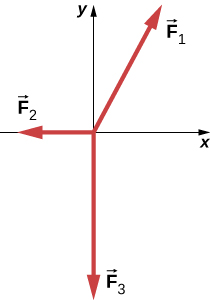Two teenagers are pulling on ropes attached to a tree. The angle between the ropes is $30.0\text{°}$. David pulls with a force of 400.0 N and Stephanie pulls with a force of 300.0 N. (a) Find the component form of the net force. (b) Find the magnitude of the resultant (net) force on the tree and the angle it makes with David’s rope.

a. ${\stackrel{\to }{F}}_{\text{net}}=660.0\stackrel{^}{i}+150.0\stackrel{^}{j}\phantom{\rule{0.2em}{0ex}}\text{N}$; b. ${F}_{\text{net}}=676.6\phantom{\rule{0.2em}{0ex}}\text{N}$ at $\theta =12.8\text{°}$ from David’s rope

### Glossary

dynamics
study of how forces affect the motion of objects and systems
external force
force acting on an object or system that originates outside of the object or system
force
push or pull on an object with a specific magnitude and direction; can be represented by vectors or expressed as a multiple of a standard force
free-body diagram
sketch showing all external forces acting on an object or system; the system is represented by a single isolated point, and the forces are represented by vectors extending outward from that point
net external force
vector sum of all external forces acting on an object or system; causes a mass to accelerate
newton
SI unit of force; 1 N is the force needed to accelerate an object with a mass of 1 kg at a rate of $1\phantom{\rule{0.2em}{0ex}}{\text{m/s}}^{2}$